Question

# Based on the average number of moles of IO3- in your samples, and the initial volume...

Based on the average number of moles of IO3- in your samples, and the initial volume of those samples, what is the solubility of Ca(IO3)2 in mol/L of the saturated solution in 0.01 M KIO3? Avg mol: 0.0000746 volume: 5mL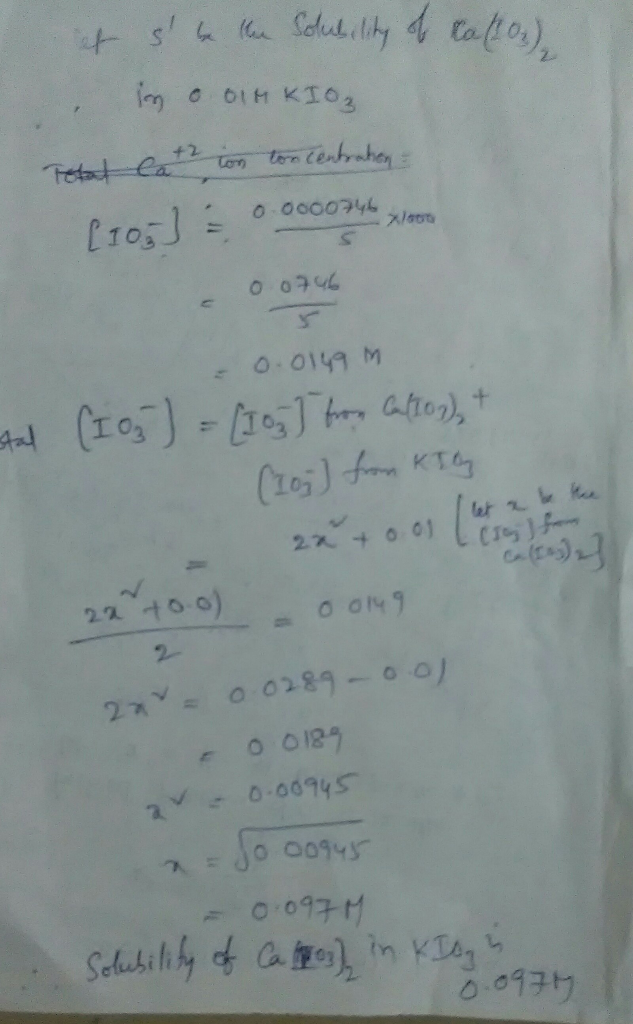#### Earn Coins

Coins can be redeemed for fabulous gifts.

Similar Homework Help Questions
• ### calculate molar solubility of Ca(IO3)2 in 0.0100M KIO3 Average lo concentration Subtract the concentration of IO...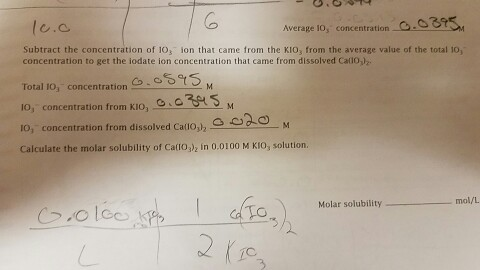calculate molar solubility of Ca(IO3)2 in 0.0100M KIO3 Average lo concentration Subtract the concentration of IO on that came from the KIO3 from the average value of the total 10i concentration to get the iodate ion concentration that came from dissolved Cadonnz. Total ro, concentration 10, concentration from Kloo lo, concentration from dissolved Callobl M Calculate the molar solubility of Ca n 0.0100 M Klo, solution. Molar solubility mol/L

• ### Cu(IO3)2 has Ksp=1.4x107. What is the molar solubility of Cu(IO3)2 (How many moles of Cu(IO3)2 can...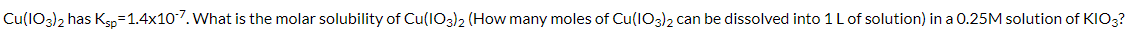Cu(IO3)2 has Ksp=1.4x107. What is the molar solubility of Cu(IO3)2 (How many moles of Cu(IO3)2 can be dissolved into 1 L of solution) in a 0.25M solution of KIO3?

• ### Molar Solubility and Solubility Product of Calcium Hydroxide Volume of saturated Ca(OH)2 solution (mL) 25.00...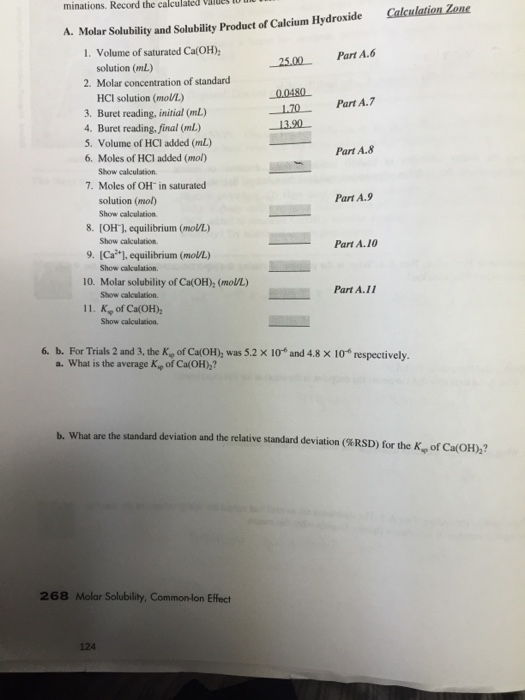Molar Solubility and Solubility Product of Calcium Hydroxide Volume of saturated Ca(OH)2 solution (mL) 25.00 Molar concentration of standard HCI solution (mol/L.) 0.0480 Buret reading, initial (mL) 1.70 Buret reading, final (mL) 13.90 Volume of HCI added (mL) Moles of HCI added (mol) Show calculation Moles of OH^- in saturated solution (mol) Show calculation [OH^-), equilibrium (mol/L) Show calculation. [Ca^2+], equilibrium (mol/L) Show calculation. Molar solubility of Ca(OH)2 (mol/L) Show calculation. Ks rho of Ca(OH)2 Show calculation. For Trials 2...

• ### A saturated solution of calcium iodate contains 2.37 g/L of Ca(IO3)2. (a) What is the solubility...

A saturated solution of calcium iodate contains 2.37 g/L of Ca(IO3)2. (a) What is the solubility of calcium iodate in mol/L? (b) What are the concentrations of Ca2+ and IO3 – ion, respectively, in the saturated solution? (c) Calculate the Ksp value for Ca(IO3)2. Part b ) Silver ion, Ag+ , readily forms complex ion, Ag(S2O3)2 3– , with thiosulfate, S2O3 2– , according to the following equation: Ag+ (aq) + 2S2O3 2– (aq) ⇌ Ag(S2O3)2 3– (aq); Kf =...

• ### The solubility product constant for Ba(IO3)2 is 1.57×10-9 at 25 oC. What is the molar concentration...

The solubility product constant for Ba(IO3)2 is 1.57×10-9 at 25 oC. What is the molar concentration of IO3- ions in a saturated solution of Ba(IO3)2? Assume an ideal solution at 25 oC. How many grams of Ba(IO3)2 (487.1 g/mol) can be dissolved in 1000 mL of pure water at 25 oC? How many grams of Ba(IO3)2 can be dissolved in 1000 mL of a 0.100 M KIO3 solution at 25  oC? Use activities for this calculation.

• ### The solubility product constant for La(IO3)3 is 1.00×10-11 at 25 oC. What is the molar concentration...

The solubility product constant for La(IO3)3 is 1.00×10-11 at 25 oC. What is the molar concentration of IO3- ions in a saturated solution of La(IO3)3? Assume an ideal solution at 25 oC. How many grams of La(IO3)3 (663.6 g/mol) can be dissolved in 900 mL of pure water at 25 oC? How many grams of La(IO3)3 can be dissolved in 900 mL of a 0.100 M KIO3 solution at 25  oC? Use activities for this calculation. Activity coefficients can be found...

• ### 1. The Molar Solubility of Ca(10), in Pure Water Temperature of the saturated solution of calcium...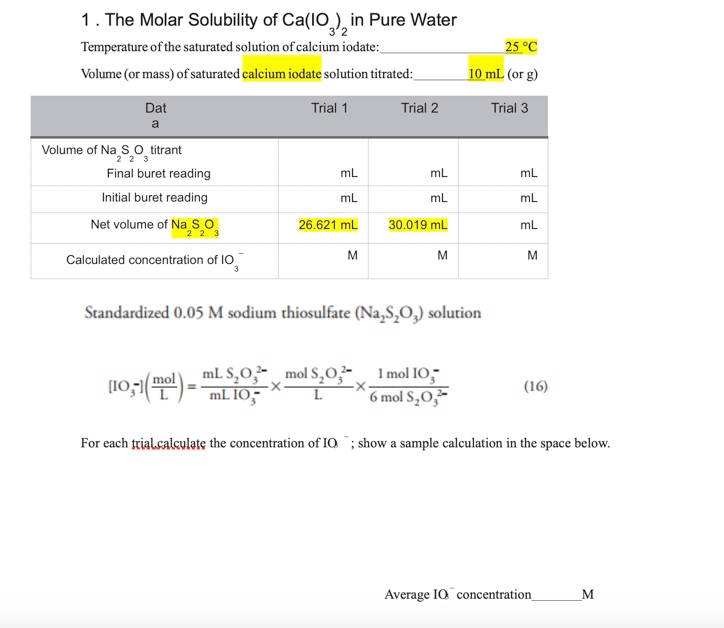1. The Molar Solubility of Ca(10), in Pure Water Temperature of the saturated solution of calcium iodate: Volume (or mass) of saturated calcium iodate solution titrated: 25 °C 10 ml (or g) Dat Trial 1 Trial 2 Trial 3 Volume of Na S o titrant Final buret reading Initial buret reading ml mL mL mL Net volume of Na So 26.621 mL 30.019 mL Calculated concentration of 10 Standardized 0.05 M sodium thiosulfate (Na,8,0,) solution (10,51(E) - TIL 10 mols.0.2...

• ### Isnt the molar solubility of Ca(OH)2 with added CaCl2 supposed to be less than the molar solubili...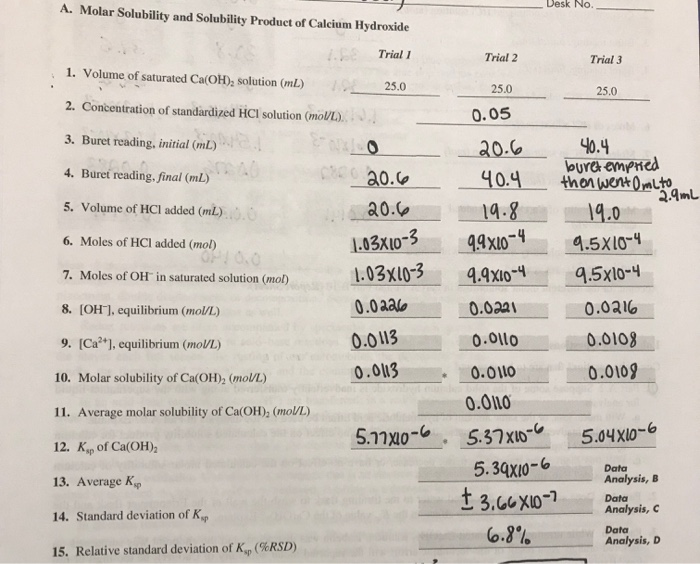isnt the molar solubility of Ca(OH)2 with added CaCl2 supposed to be less than the molar solubility of Ca(OH)2 by itself? where did I mess up? A. Molar Solubility and Solubility Product of Calcium Hydroxide メーーー Desk No. Trial 1 Trial 2 Trial 3 1. Volume of saturated CalOHD, solution (ml) 2. Concentration of standardized HCI solution (mol/L) 3. Buret reading, initial (mL) . 4. Buret reading,final(mL) 5. Volume of HCI added (mL) 6. Moles of HCl added (mol) 7....

• ### A determination the molar solubility and the K_sp calcium hydroxide was completed according to the experimenta...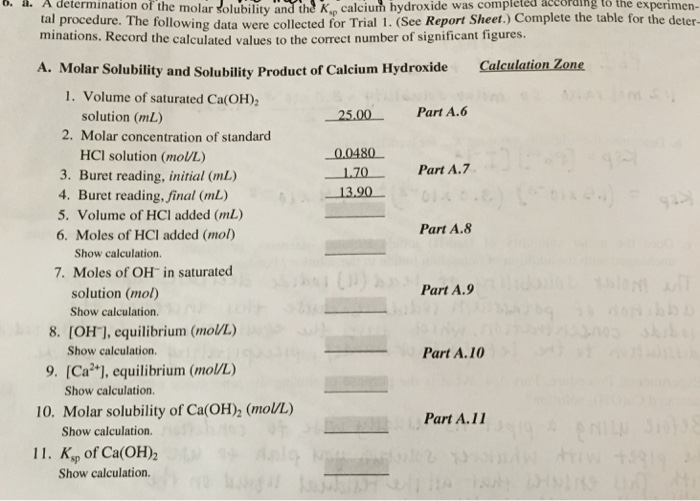A determination the molar solubility and the K_sp calcium hydroxide was completed according to the experimental procedure. The following data were collccicd for Trial 1 (Sec Repon sheet. minations. Record the calculated values to the correct number of significant ligures. A. Molar Solubility and Solubility Product of Calcium Hydroxide 1.Volume of saturated Ca(OH)_2 solution (mL) 2.Molar concentration of standard HCI solution (mol/L) 3.Buret reading, initial (mL) 4.Buret reading, final (mL) 5.Volume of HCI added (mL) 6.Moles of HCI added (mol)...

• ### Part A and Part B. Also question #3-post lab. (its circled) A. Molar Solubility and Solubility Product of Calcium Hydroxide Trial I Trial 3 Trial 2 25.0 1. Volume of saturated Ca(OH), solution (m...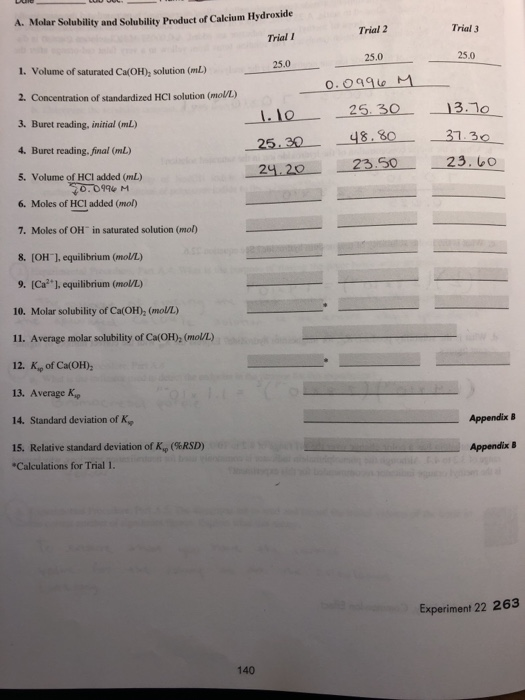Part A and Part B. Also question #3-post lab. (its circled) A. Molar Solubility and Solubility Product of Calcium Hydroxide Trial I Trial 3 Trial 2 25.0 1. Volume of saturated Ca(OH), solution (mL) 2. Concentration of standardized HCl solution (molU/L) 3. Buret reading, initial (mL 4. Buret reading, final (mL) 5. Volume of HCI added (mL) 6. Moles of HCI added (mol) 7. Moles of OH" in saturated solution (mol) 8. (OH1, equilibrium (mol/L) 9. (Ca2 ], equilibrium (mol/L)...